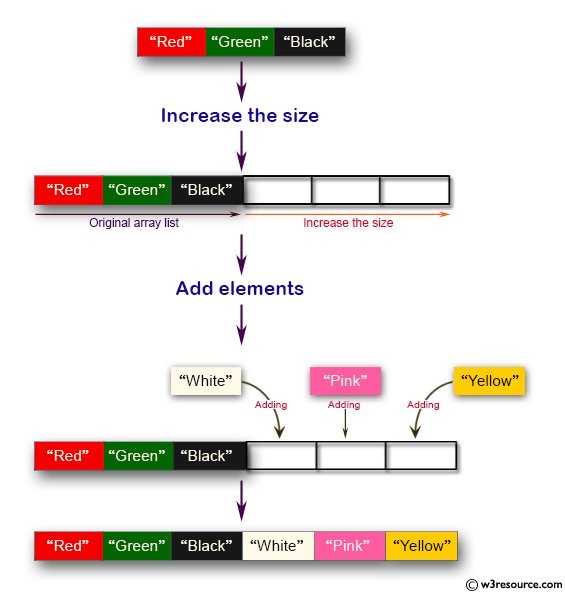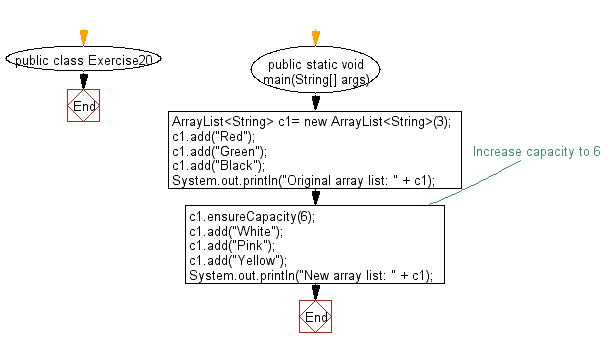﻿ Java Collection exercises: Increase the size of an array list - w3resource# Java Collection, ArrayList Exercises: Increase the size of an array list

## Java Collection, ArrayList Exercises: Exercise-20 with Solution

Write a Java program to increase the size of an array list.

Pictorial Presentation:Sample Solution:-

Java Code:

``````import java.util.ArrayList;
import java.util.Collections;
public class Exercise20 {
public static void main(String[] args) {
ArrayList<String> c1= new ArrayList<String>(3);
System.out.println("Original array list: " + c1);
//Increase capacity to 6
c1.ensureCapacity(6);
System.out.println("New array list: " + c1);
}
}
``````

Sample Output:

```Original array list: [Red, Green, Black]
New array list: [Red, Green, Black, White, Pink, Yellow]
```

Flowchart:Java Code Editor:

What is the difficulty level of this exercise?

Test your Programming skills with w3resource's quiz.

﻿

## Java: Tips of the Day

countOccurrences

Counts the occurrences of a value in an array.

Use Arrays.stream().filter().count() to count total number of values that equals the specified value.

```public static long countOccurrences(int[] numbers, int value) {
return Arrays.stream(numbers)
.filter(number -> number == value)
.count();
}
```

Ref: https://bit.ly/3kCAgLb